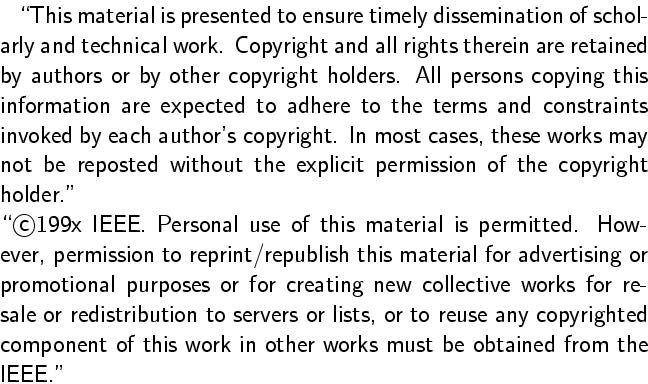Gunther Reißig.
Semi-Implicit Differential-Algebraic Equations Constitute a Normal Form.
IEEE Trans. CAS, Part I, vol. 42, no. 7, July 1995, pp. 399-402.
Full text. (Definitive publication; restricted access.)
Full text. (Free access.)Abstract:
Continuously differentiable functions, the total derivative or a partial derivative of which is of constant rank, play a part in many engineering problems. One usually exploits this property of constancy of rank by applying the Rank Theorem. However, in case only a partial derivative is of constant rank, which is the natural situation for functions involved in Differential-Algebraic Equations (DAEs), this theorem does not apply immediately. In this note, we generalize known results to the latter case. More precisely, we give a parametrized version of the Rank Theorem and results on functional dependence and present a normal form for a class of nonlinear equations. Although these results are general in nature, the fundamental conclusion with respect to DAEs is that here the normal form exactly corresponds to semi-implicit DAEs. We also generalize results from the solution theory of DAEs in case differential geometric techniques fail to apply. Such DAEs occur, for example, in the analysis of certain circuits.
BibTeX entry:
```@article{Reissig95b,
AUTHOR = {Rei{\ss}ig, Gunther},
TITLE = {Semi-implicit differential-algebraic equations constitute a normal form},
JOURNAL = {IEEE Trans. Circuits Systems I Fund. Theory Appl.},
VOLUME = {42},
YEAR = {1995},
NUMBER = {7},
PAGES = {399-402},
doi = {10.1109/81.401157}
}
```

Impressum und Haftungsausschluß